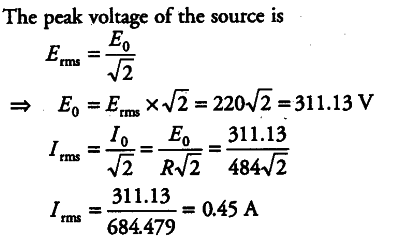# A light bulb is rated 100 W for 220 V

A light bulb is rated 100 W for 220 V AC supply of 50 Hz. Calculate
(i) resistance of the bulb.
(ii) the rms current through the bulb.

(i) Power, P = VI
P = V x V/R
R = V^{2} / P = 220 x 220 / 100 = 48400 / 100 = 484

(ii)